# Decomposition of Force（Use Trigonometric Ratios）

When we dealt with decomposition of force in the past, the magnitude of the partial force was calculated using the ratio of the sides of a right triangle.

https://www.yukimura-physics.com/entry/dyn-f17

However, the ratios of the sides are at most 30°, 45°, and 60°, and other angles are not known.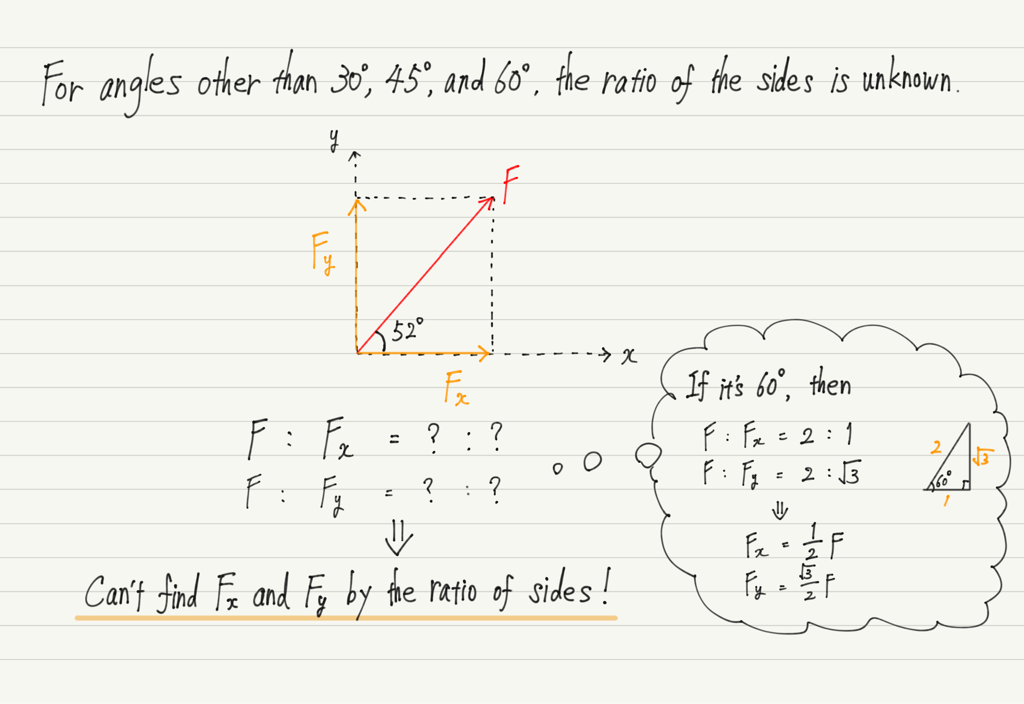So, in this lecture, I will show you how to find the decomposed force that can be used for any angle!

## Using trigonometric ratios

The way to find the decomposed force of any angle is to use trigonometric ratios!

It is not a difficult application. Let’s start with a review of trigonometric ratios.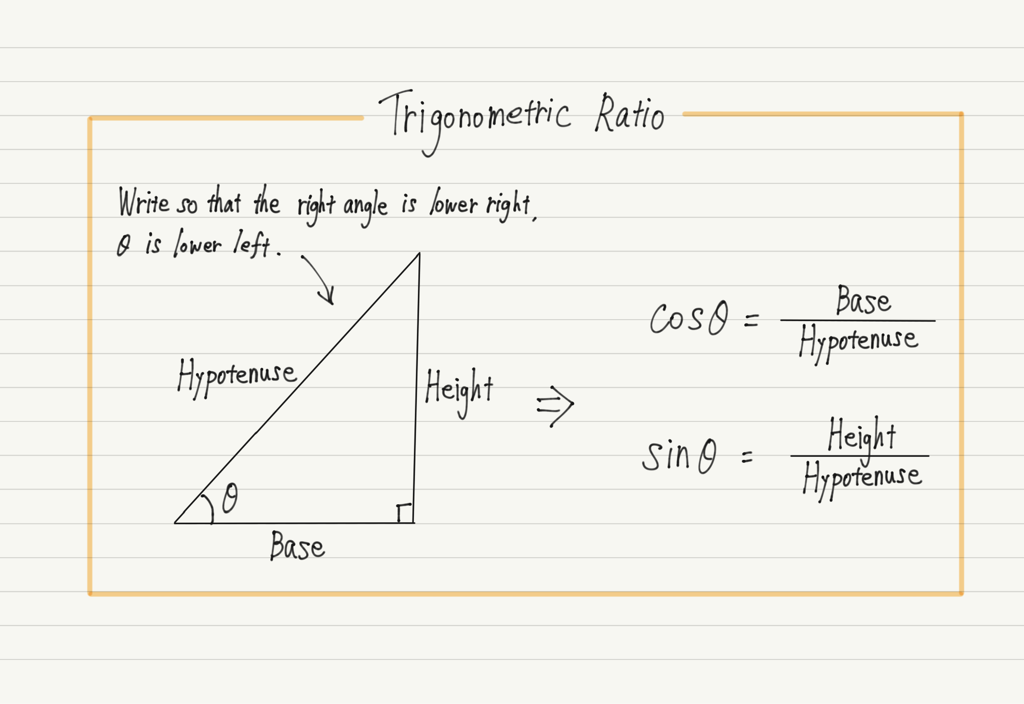By applying this to the decomposition of force and transforming the equation, we obtain the x– and y-components.
Let’s try it in practice using triangles with appropriate angles.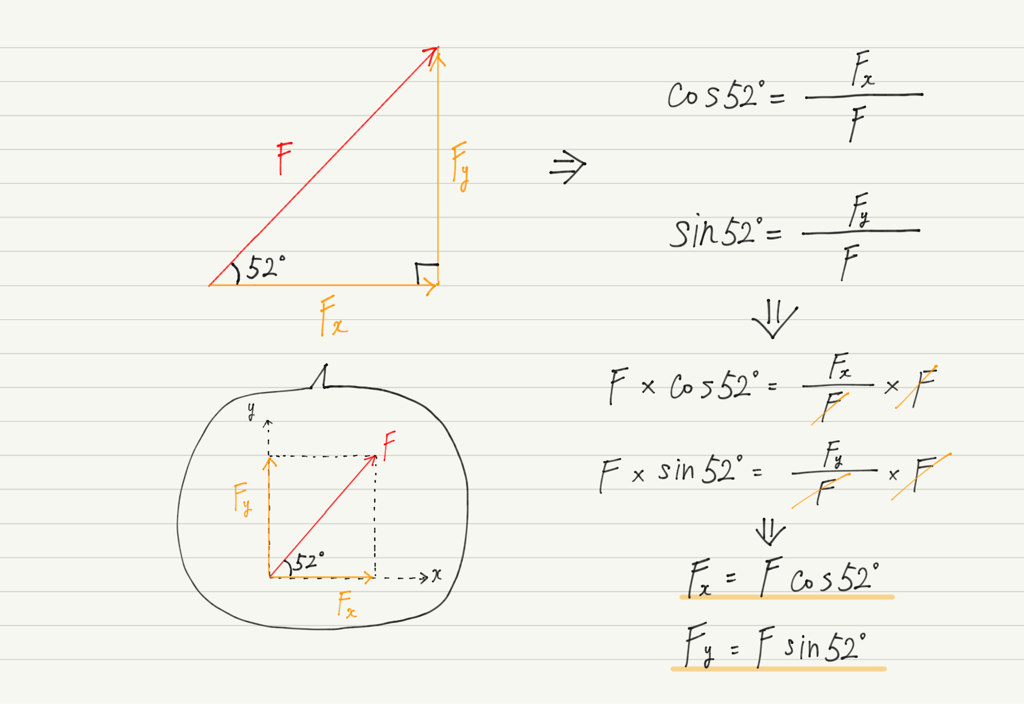We don’t know the ratios of the sides of a 52° triangle, but we can easily find the values of sin52° and cos52° by typing them into a calculator.
Of course, 52° is just one example; it is possible to find the decomposed force by multiplying sin and cos to the oblique force at any other angle.

## Which is sin and which is cos?

The decomposition of force is not always in the horizontal and vertical directions.
For example, gravity acting on an object on a slope decomposes in the direction of the slope and in the direction perpendicular to it.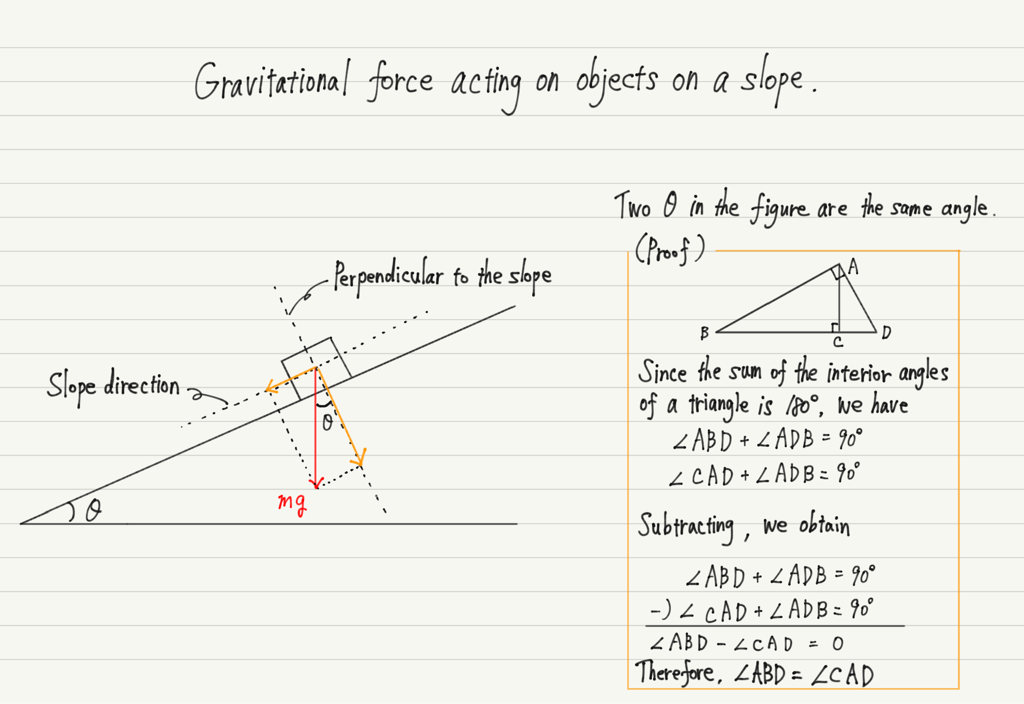Now, to find the decomposed force, we can multiply the original force mg by either sinθ or cosθ.
Can you quickly determine which is mgsinθ and which is mgcosθ?

Of course, if you think of the triangle in a different orientation, you will understand.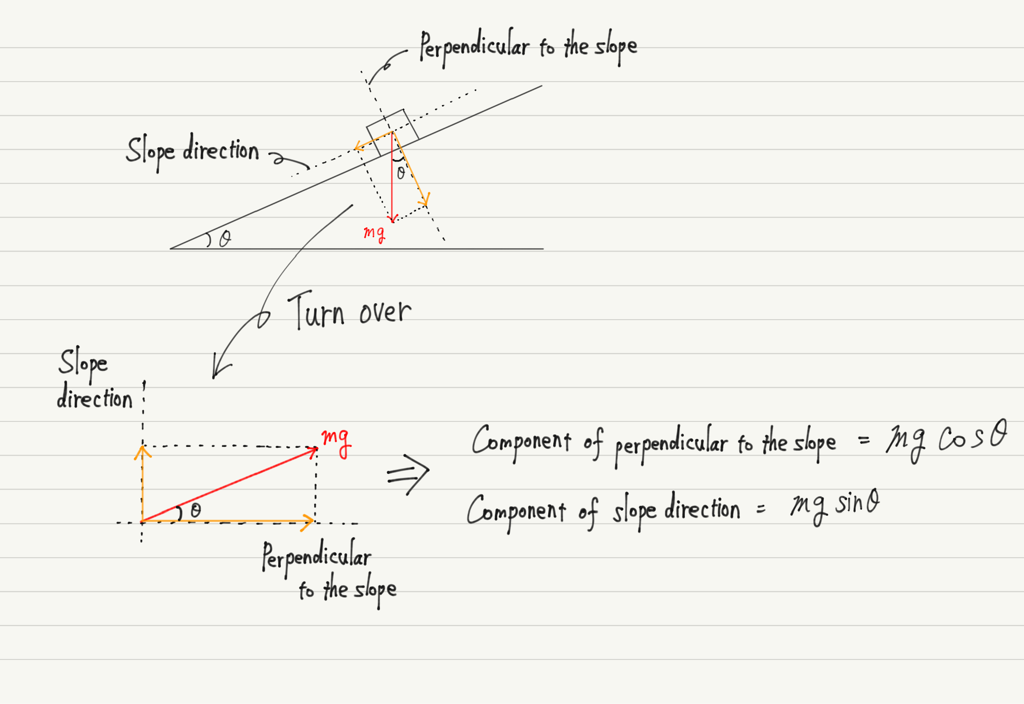However, it is tedious to change the orientation and think about it every time.
Is there any regularity?

Let’s find the regularity through examples.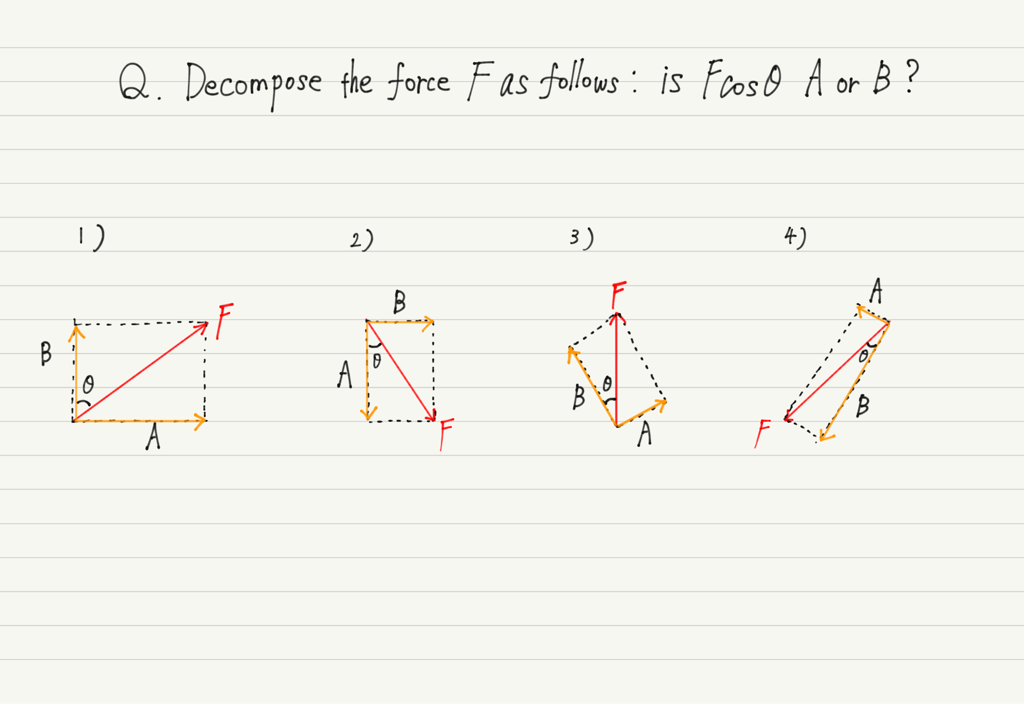Think for yourself first, and then scroll down for the answer.

The answers to the questions are
1) B
2) A
3) B
4) B.

Now, have you figured out the regularity of Fsinθ and Fcosθ?

Actually, it’s easy to spot them this way!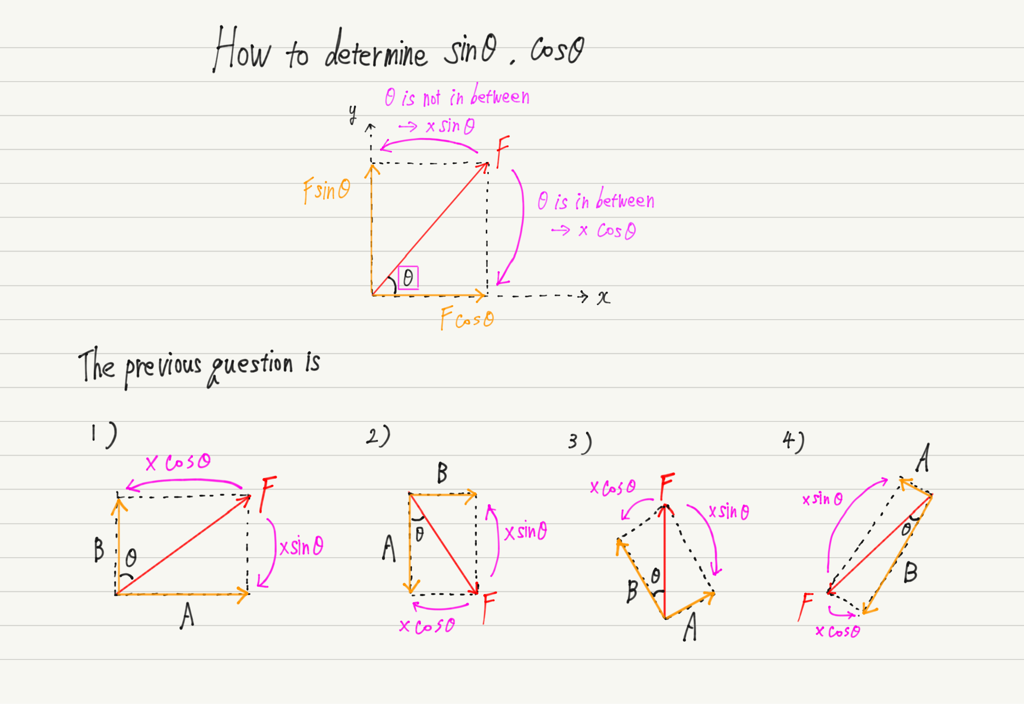Once you have this down, you won’t have to draw triangles or change their orientation every time!

## Summary of this lecture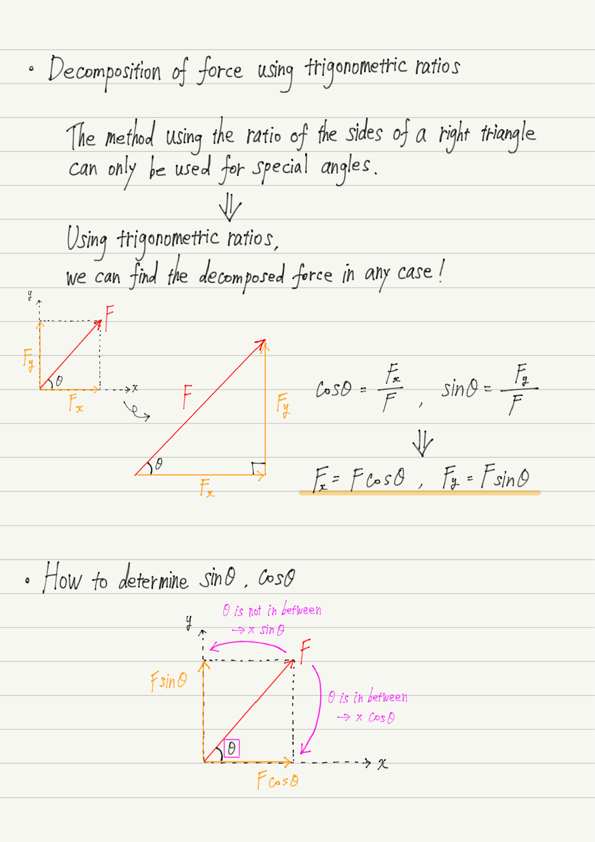Many people react negatively when trigonometric ratios appear, but in fact, they are not that difficult.
You will get used to it as you solve many problems, so don’t be afraid to try problems involving trigonometric ratios!

error: Content is protected !!